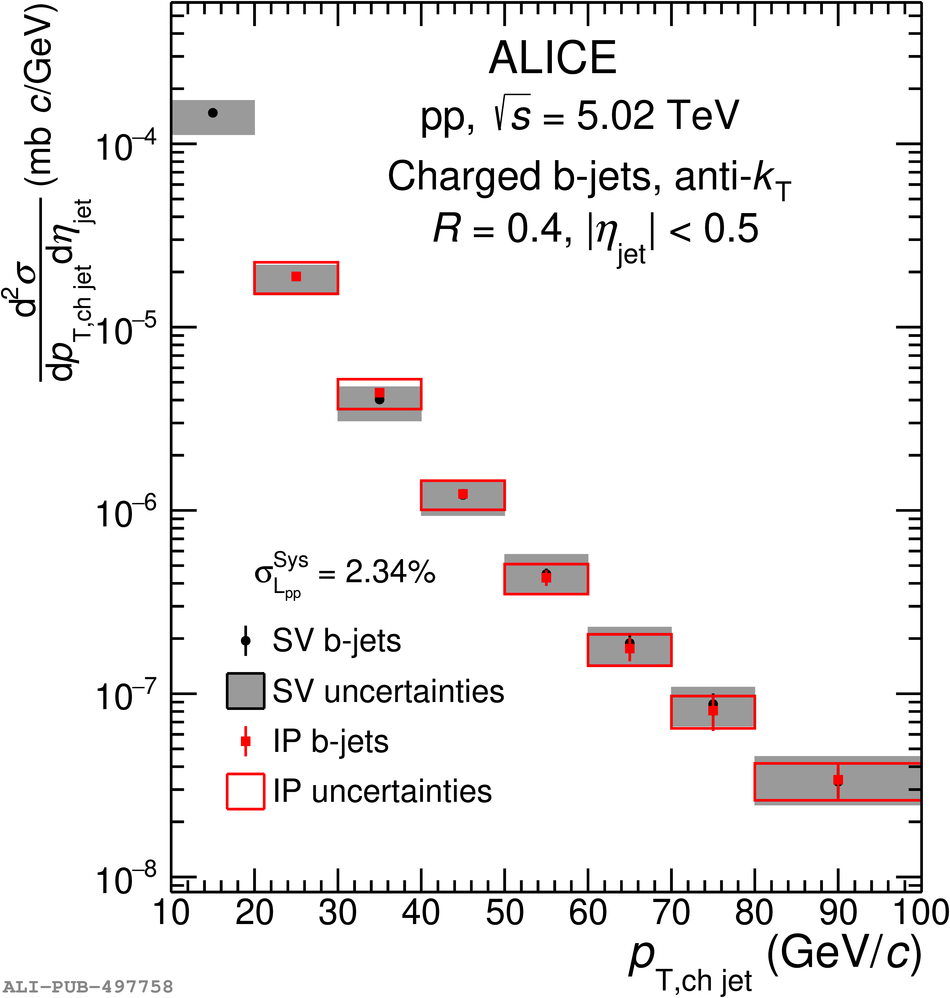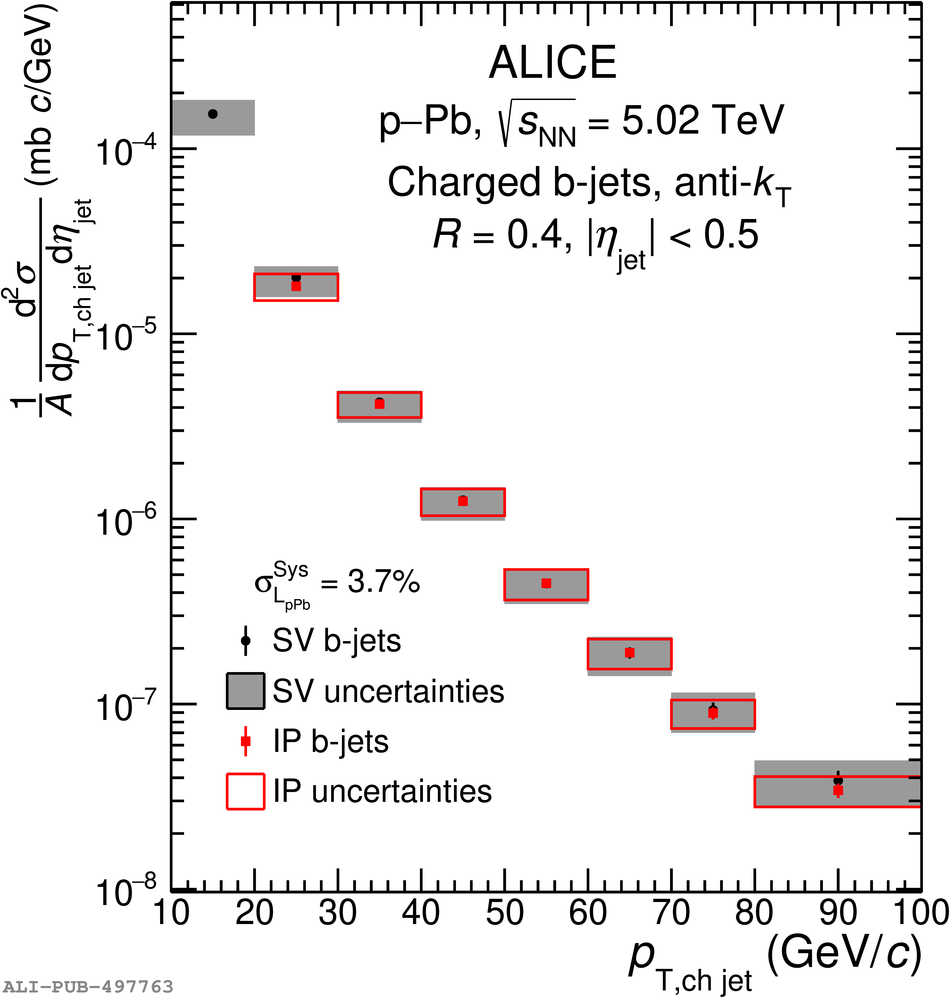# Figure 11

 Comparison of the \pT differential production cross section of charged-particle anti-$k_{\rm T}$ $R=0.4$ b jets measured in pp and \pPb collisions at $\snn=5.02$\,TeV using the IP and SV methods. Systematic and statistical uncertainties are shown as boxes and error bars respectively. The additional common normalization uncertainty due to luminosity is denoted $\sigma_{\mathcal{L}}^{\rm Sys}$ and it is quoted separately.